Get instant live expert help with Excel or Google Sheets“My Excelchat expert helped me in less than 20 minutes, saving me what would have been 5 hours of work!”

#### Post your problem and you’ll get expert help in seconds.

Your message must be at least 40 characters
Our professional experts are available now. Your privacy is guaranteed.

# How to solve quadratic equation in excel

We can solve quadratic equations in excel by inputting an equation that contains our variable (e.g. X), then we would define X using a formula. Thereafter, we would use this quadratic formula to solve for X using some given roots of the equation. This tutorial will teach use how to solve quadratic equation in excel.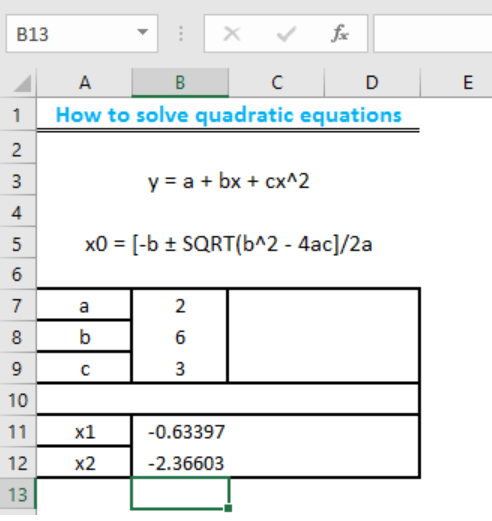Figure 1: Solving quadratic equations

## Data for Solving Quadratic Equation

• To do this, we will type in our quadratic equation `y = a + bx + cx^2` and also define the root of the variable “X” by typing this quadratic formula `x0 = [-b ± SQRT(b^2 - 4ac]/2a`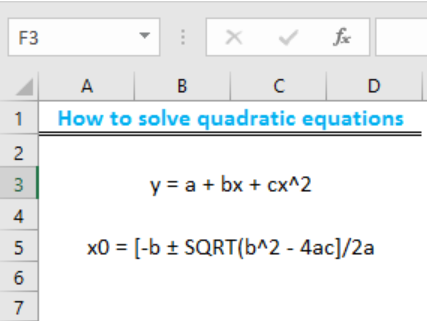Figure 2: Quadratic formula

• We will now prepare a table for the roots of “X” which are “x1” and “x2”, and ascribing values for the variables in the equation of “X” which are “a, b, and c”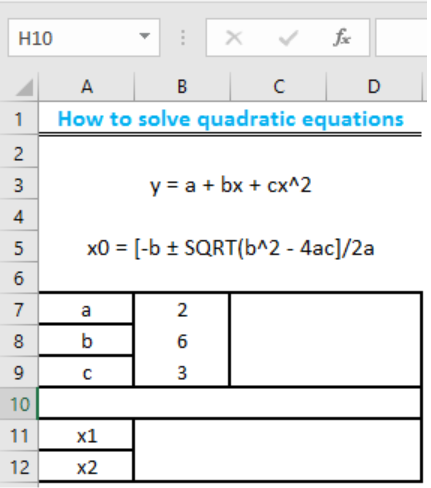Figure 3: Table of roots

• We will type this formula into Cell B11 `=(-B8+SQRT(B8^2-4*B7*B9))/(2*B7)`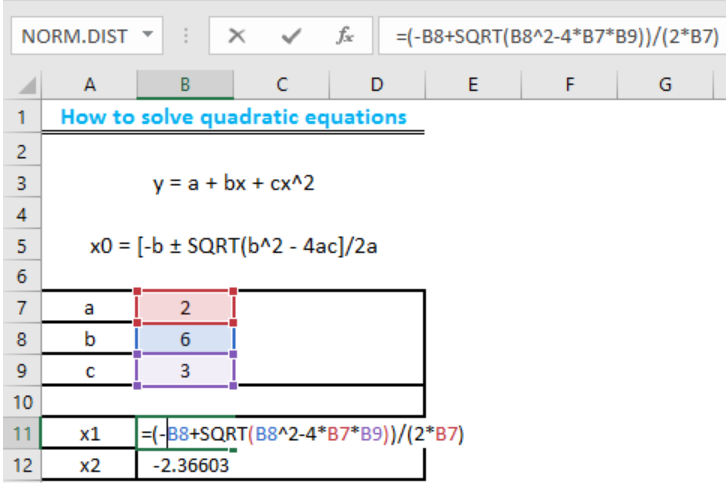Figure 4a: Formula for the positive root of the equation

• We will now press the enter key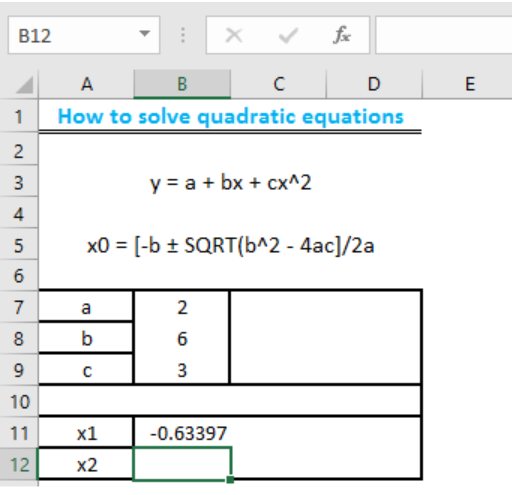Figure 4b: Answer for the positive root of equation

• We will now repeat the same operation for “x2” by copying, pasting and changing “+” sign into a ““minus sign in the formula in Cell B12 as =(-B8-SQRT(B8^2-4*B7*B9))/(2*B7)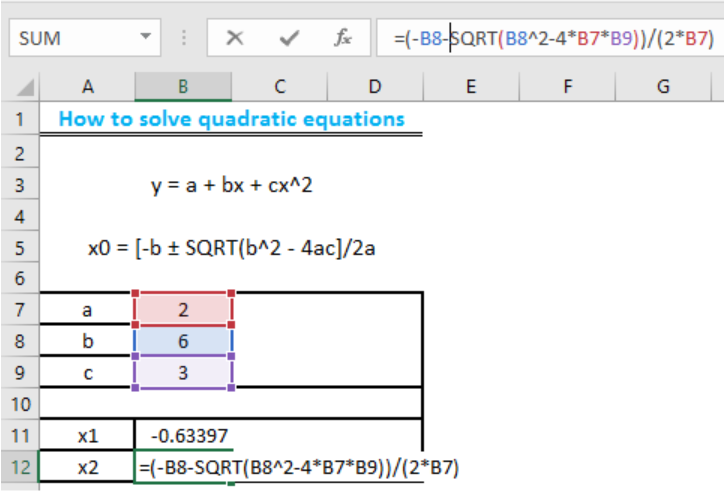Figure 5a: Formula for the negative root of the equation

• We will now press the enter key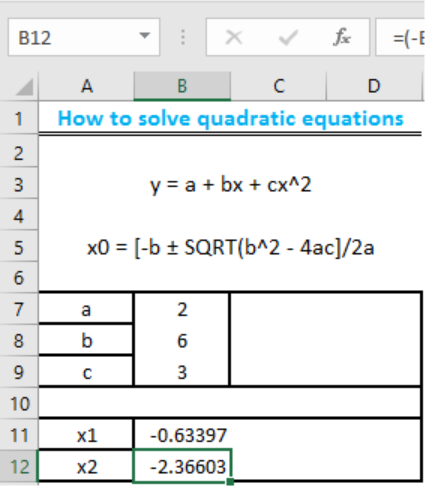Figure 5b: Solved quadratic equation

## Instant Connection to an Expert through our Excelchat Service

Most of the time, the problem you will need to solve will be more complex than a simple application of a formula or function. If you want to save hours of research and frustration, try our live Excelchat service! Our Excel Experts are available 24/7 to answer any Excel question you may have. We guarantee a connection within 30 seconds and a customized solution within 20 minutes.